# Making a queue using an array in C

May 26, 2017 57827

### Previous:

The previous article was all about introducing you to the concepts of a queue. In this article, we will code up a queue and all its functions using an array.

As we know that we can’t change the size of an array, so we will make an array of fixed length first (this will be the maximum length of our queue) and then implement the queue on it.

#include <stdio.h>
#define MAXSIZE 10

int queue[MAXSIZE];


We will use three pointers to implement the queue using an array, ‘size’, ‘front’ and ‘rear’. ‘front’ and ‘rear’ will simply store the indices of the front and rear elements respectively. We will use ‘size’ to store the current size of the queue.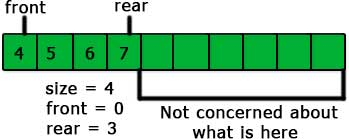In the above picture, the value of ‘size’ is 4 because there are four elements in the queue and the value of ‘rear’ and ‘front’ are 3 and 4 respectively because these are the indices of the rear and front elements. Also, we don’t care about the elements present outside the range of the queue.

#include <stdio.h>
#define MAXSIZE 10

int queue[MAXSIZE];

int front = -1;
int rear = -1;
int size = -1;


We have made the values of ‘front’, ‘rear’ and ‘size’ -1 because the queue is not yet initialized. We will change these values according to our need after the initialization of the queue.

The next and the most important operations on a stack are enqueue and dequeue. So, let’s create them.

### enqueue

We just need to add an element at the index ‘rear+1’ and increase the value of the ‘rear’ and size by 1 for the enqueue operation.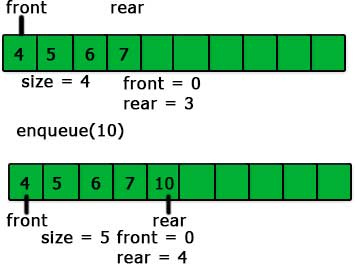There is one more case in the enqueue operation when the rear is the last element of the array and the queue is not full. It is shown in the picture given below.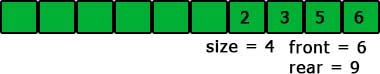Here, the new element on the enqueue operation will be added to the first element of the array i.e., the element having 0 index.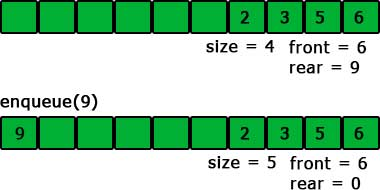You can see that the new element is added at the beginning of the array and the pointer ‘rear’ is changed to 0.

Let’s write the code for enqueue operation using the concepts given above.

void enqueue(int value)
{
if(size<MAXSIZE)
{
if(size<0)
{
queue = value;
front = rear = 0;
size = 1;
}
else if(rear == MAXSIZE-1)
{
queue = value;
rear = 0;
size++;
}
else
{
queue[rear+1] = value;
rear++;
size++;
}
}
else
{
printf("Queue is full\n");
}
}


if(size<MAXSIZE) – We are just making sure that the queue is not full

if(size<0) – The list is not initialized yet. We will initialize the list by making the both ‘front’ and ‘rear’ 0 and ‘size’ 1 and then we will give the value to the first element of the list.
queue = value; front = rear = 0; size = 1;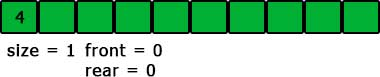else if(rear == MAXSIZE-1) – This is the case when the ‘rear’ is the last element pf the array. We will add a new element at the index 0.
queue = value; rear = 0; size++;

else {  queue[rear+1] = value;  rear++;  size++; }

This is the normal case and we have coded accordingly. We are giving the value to the element with index ‘rear+1’ and then increasing both ‘rear’ and ‘size’ by 1.

### dequeue

The ‘dequeue’ operation is very simple using the array. We just need to decrease the ‘size’ by 1 and increase the ‘front’ by 1. That’s it.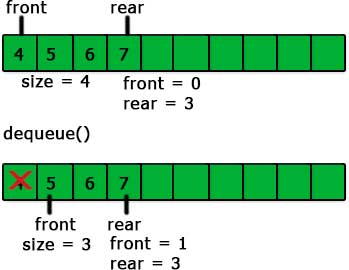int dequeue()
{
if(size<0)
{
printf("Queue is empty\n");
}
else
{
size--;
front++;
}
}


Thus, the overall code is:

#include <stdio.h>
#define MAXSIZE 10

int queue[MAXSIZE];

int front = -1;
int rear = -1;
int size = -1;

int isempty()
{
return size<0;
}

int isfull()
{
return size == MAXSIZE;
}

void enqueue(int value)
{
if(size<MAXSIZE)
{
if(size<0)
{
queue = value;
front = rear = 0;
size = 1;
}
else if(rear == MAXSIZE-1)
{
queue = value;
rear = 0;
size++;
}
else
{
queue[rear+1] = value;
rear++;
size++;
}
}
else
{
printf("Queue is full\n");
}
}

int dequeue()
{
if(size<0)
{
printf("Queue is empty\n");
}
else
{
size--;
front++;
}
}

int Front()
{
return queue[front];
}

void display()
{
int i;
if(rear>=front)
{
for(i=front;i<=rear;i++)
{
printf("%d\n",queue[i]);
}
}
else
{
for(i=front;i<MAXSIZE;i++)
{
printf("%d\n",queue[i]);
}
for(i=0;i<=rear;i++)
{
printf("%d\n",queue[i]);
}
}
}

int main()
{
enqueue(4);
enqueue(8);
enqueue(10);
enqueue(20);
display();
dequeue();
printf("After dequeue\n");
display();
enqueue(50);
enqueue(60);
enqueue(70);
enqueue(80);
dequeue();
enqueue(90);
enqueue(100);
enqueue(110);
enqueue(120);
printf("After enqueue\n");
display();
return 0;
}


## Comparing queue using linked list and queue using array

• Array implementation is easy.
• Array implementation has limited capacity because of using a fixed size array.
• Array implementation requires less space.
• Special behavior is needed when the rear reaches the end of the array.

Liked the post?
Developer and founder of CodesDope.